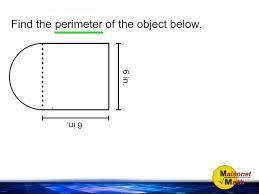# How Much Do You Know About Areas Of Rectangles?

10 Questions | Total Attempts: 132SettingsThe area of a polygon is the number of square units inside the polygon. To understand the difference between perimeter and area, think of perimeter as the length of fence needed to enclose the yard, whereas area is the spaceinside the yard.

Related Topics
• 1.
To find the area of a rectangle, multiply the .....?
• A.

Length by the width

• B.

Breath by the length

• C.

Width by the breath

• D.

Length by the breath

• 2.
A square is a rectangle with how many equal sides?
• A.

Two unequal sides

• B.

Four equal sides

• C.

Three sides

• D.

Four curved sides

• 3.
The word rectangle comes from the ..... rectangulus?
• A.

Latin

• B.

Greek

• C.

Spanish

• D.

Yoruba

• 4.
A rectangle is a special case of a ......?
• A.

Cuboid

• B.

Polygon

• C.

Parallelogram

• D.

Octagon

• 5.
Who defines a rectangle more generally as any quadrilateral with axes of symmetry through each pair of opposite sides?
• A.

De Villiers

• B.

McGregory

• C.

Newton

• D.

Einstein

• 6.
A rectangle with four sides of equal length is a .....?
• A.

Circle

• B.

Square

• C.

Triangle

• D.

Oval

• 7.
What is the term occasionally used to refer to a non-square rectangle?
• A.

Oblate

• B.

Prolate

• C.

Rectangle

• D.

Oblong

• 8.
A crossed rectangle is a crossed (self-intersecting) quadrilateral which consists of how many opposite sides of a rectangle along with the two diagonals?
• A.

Two

• B.

Three

• C.

Four

• D.

Five

• 9.
Rectangle is a parallelogram with diagonals of equal ....?
• A.

Length

• B.

Width

• C.

• D.

Height

• 10.
A convex quadrilateral has how many features?
• A.

Two

• B.

One

• C.

Four

• D.

Five